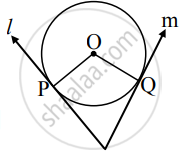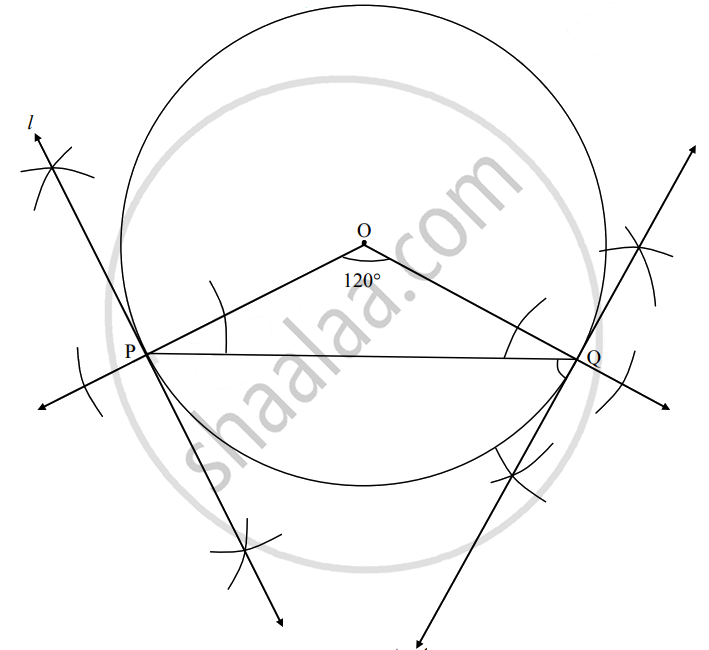# Draw a circle of radius 4.2 cm. Draw arc PQ measuring 120°. Draw a tangent to the circle from point P and point Q - Geometry

Diagram

Draw a circle of radius 4.2 cm. Draw arc PQ measuring 120°. Draw a tangent to the circle from point P and point Q

#### SolutionAnalysis:
Let O be the centre of the circle.

Here, ∠POQ = m(arc PQ)     ......[Definition of measure of minor arc]

∴ On drawing ∠POQ = 120°, we get an arc

PQ measuring 120°.

line l and line m are tangents to the circle.

line l ⊥ seg OP and line m ⊥ seg OQ   ......[Tangent theorem]

∴ To get tangents l and m, we draw perpendiculars to seg OP and seg OQ at points P and Q respectively.Steps of construction:

1. Draw a circle of radius 4.2 cm with centre O.
2. Draw rays OP and OQ such that ∠POQ = 120°. (Points P and Q must be on the circle.)
3. Draw line l ⊥ ray OP at point P
4. Draw line m ⊥ ray OQ at point Q.
Line l and m are the required tangents.
Concept: Construction of a Tangent to the Circle at a Point on the Circle
Is there an error in this question or solution?

Share